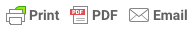# 1.2 Word Problemswww.MyHSMath.com

1.2 - Pre-Word Problems Worksheet

Part 1 - Convert each to it's algebraic equivalent.

1)  The difference of 7 and 5                            2)  x decreased by 17

3)  The quotient of 12 and 6                             4)  Half of 16

5)  x increased by 5                                            6)  The product of x and 3

7)  3 squared                                                        8)  The sum of x and 7

9)  Twice y                                                           10)  Six plus seven is x

11)  The quotient of 12 and x                             12)  a cubed

Part 2  Solve each expression

13) One third of 27                                                14) 4 squared

15) 18 is reduced by 6                                           16) 7 doubled

17) The quotient of 8 and 2                                  18) The product of 5 and 3

19) 12 divided by 3                                                   20)  20 increased by 3

21)  10 decreased by 7                                            22)  5 tripled

23)  6 less than 10                                                   24)   2 times 4

www.MyHSMath.com